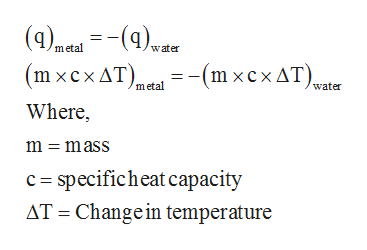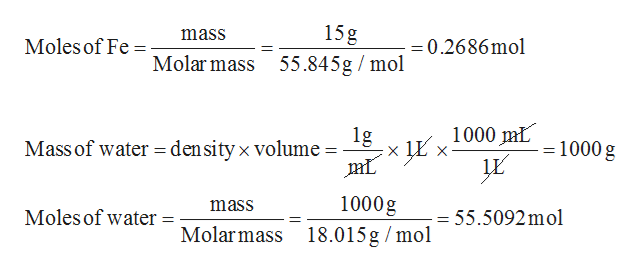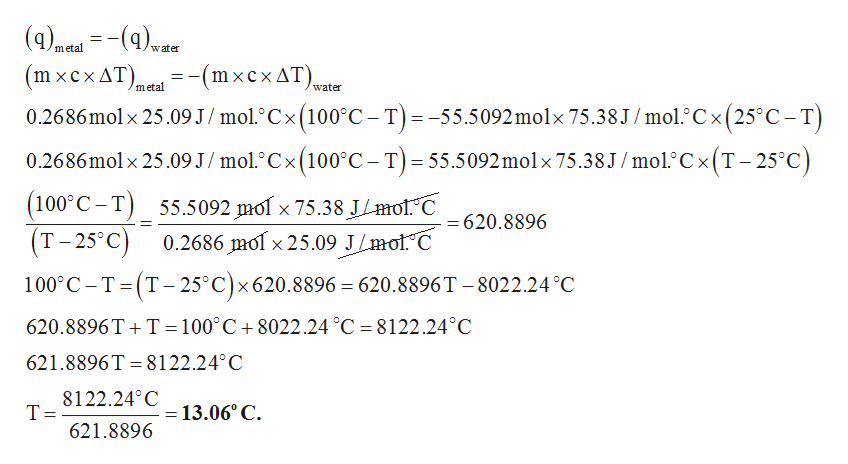# 4. A 15 g piece of iron [cp = 25.09 J/(mol * °C)] is heated to a temperature of 100°C and placed into a bucket containing 1.0 L of water [cp = 75.38 J/(mol * °C)] at 25°C. What is the final temperature of the piece of iron and the water.

Question
2 views

4. A 15 g piece of iron [cp = 25.09 J/(mol * °C)] is heated to a temperature of 100°C and placed into a bucket containing 1.0 L of water [cp = 75.38 J/(mol * °C)] at 25°C. What is the final temperature of the piece of iron and the water.

check_circle

Step 1

The concept behind this question is the heat released by the metal is absorbed by the water. This can be represented by an equation as given below.help_outlineImage Transcriptionclose(4)aa()er (mxсxAT). - (m хсхдT).. Where metal water -(m xcx AT), metal water m = mass c specificheat capacity AT Change in temperature fullscreen
Step 2

The negative sign is indicating that the heat is released. The specific heat capacity is given in the unit of J/mol 0C, so mass should be converted into moles.

The calculation of moles are given below.help_outlineImage Transcriptionclose15g mass Moles of Fe =0.2686mol Molar mass 55.845g mol 1000 mL 1g density x volume Mass of water = 1000 g 1000g mass Moles of water = 55.5092mol Molar mass 18.015g mol fullscreen
Step 3

Suppose, the final temperature for both iron and water is T. Then, by plugging all the val...help_outlineImage Transcriptionclose(4)mea(q)w metal water -(mxcxAT) 0.2686mol x 25.09 J/ mol.°Cx (100°C- T) mxсxдT)_. metal water -55.5092 molx 75.38J / mol.° C x (25° C- T) 0.2686mol x 25.09 J / mol.°Cx (100°C- T)= 55.5092molx 75.38 J / mol° C x (T - 25°C) (100°C-T) T-25°C) 55.5092 mol x 75.38 J/mof C 0.2686 mol x 25.09 J/mol^C = 620.8896 100 C T (T-25°C)x 620.8896 = 620.8896 T -8022.24 °C 620.8896T T= 100°C +8022.24 °C 8122.24°C 621.8896T 8122.24° C = 8122.240 C Т- 621.8896 13.06° C fullscreen

### Want to see the full answer?

See Solution

#### Want to see this answer and more?

Solutions are written by subject experts who are available 24/7. Questions are typically answered within 1 hour.*

See Solution
*Response times may vary by subject and question.
Tagged in

### Physical Chemistry